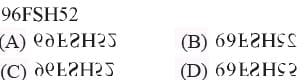# Olympiad Test: Water Images -2

Test Description

## 10 Questions MCQ Test Science Olympiad Class 7 | Olympiad Test: Water Images -2

Olympiad Test: Water Images -2 for Class 7 2023 is part of Science Olympiad Class 7 preparation. The Olympiad Test: Water Images -2 questions and answers have been prepared according to the Class 7 exam syllabus.The Olympiad Test: Water Images -2 MCQs are made for Class 7 2023 Exam. Find important definitions, questions, notes, meanings, examples, exercises, MCQs and online tests for Olympiad Test: Water Images -2 below.
 1 Crore+ students have signed up on EduRev. Have you?
Olympiad Test: Water Images -2 - Question 1

### Directions: In each of the following questions, you are given a combination of alphabets and/or numbers followed by four alternatives (A), (B), (C) and (D).  Q. Choose the alternative which closely resembles the water-image of the given combination.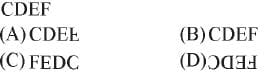Olympiad Test: Water Images -2 - Question 2

### Directions: In each of the following questions, you are given a combination of alphabets and/or numbers followed by four alternatives (A), (B), (C) and (D).  Q. Choose the alternative which closely resembles the water-image of the given combination.Olympiad Test: Water Images -2 - Question 3

### Directions: In each of the following questions, you are given a combination of alphabets and/or numbers followed by four alternatives (A), (B), (C) and (D).  Q. Choose the alternative which closely resembles the water-image of the given combination.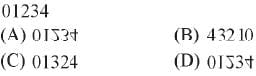Olympiad Test: Water Images -2 - Question 4

Directions: In each of the following questions, you are given a combination of alphabets and/or numbers followed by four alternatives (A), (B), (C) and (D).

Q. Choose the alternative which closely resembles the water-image of the given combination.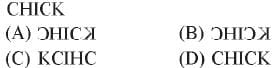Olympiad Test: Water Images -2 - Question 5

Directions: In each of the following questions, you are given a combination of alphabets and/or numbers followed by four alternatives (A), (B), (C) and (D).

Q. Choose the alternative which closely resembles the water-image of the given combination.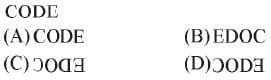Olympiad Test: Water Images -2 - Question 6

Directions: In each of the following questions, you are given a combination of alphabets and/or numbers followed by four alternatives (A), (B), (C) and (D).

Q. Choose the alternative which closely resembles the water-image of the given combination.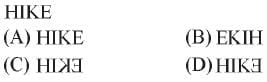Olympiad Test: Water Images -2 - Question 7

Directions: In each of the following questions, you are given a combination of alphabets and/or numbers followed by four alternatives (A), (B), (C) and (D).

Q. Choose the alternative which closely resembles the water-image of the given combination.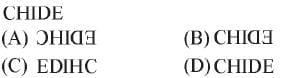Olympiad Test: Water Images -2 - Question 8

Directions: In each of the following questions, you are given a combination of alphabets and/or numbers followed by four alternatives (A), (B), (C) and (D).

Q. Choose the alternative which closely resembles the water-image of the given combination.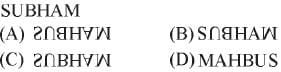Olympiad Test: Water Images -2 - Question 9

Directions: In each of the following questions, you are given a combination of alphabets and/or numbers followed by four alternatives (A), (B), (C) and (D).

Q. Choose the alternative which closely resembles the water-image of the given combination.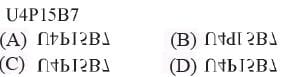Olympiad Test: Water Images -2 - Question 10

Directions: In each of the following questions, you are given a combination of alphabets and/or numbers followed by four alternatives (A), (B), (C) and (D).

Q. Choose the alternative which closely resembles the water-image of the given combination.